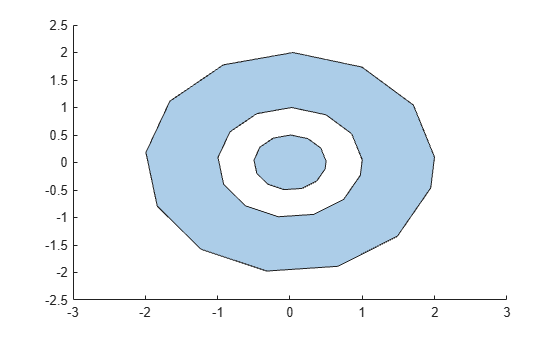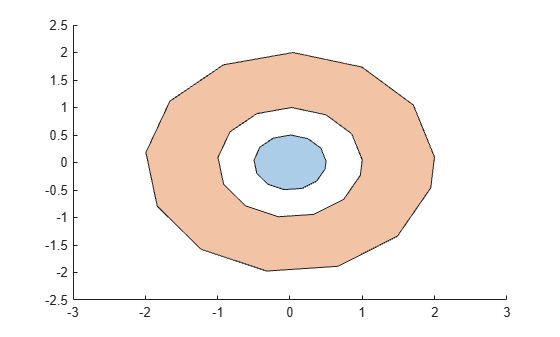sortregions

Sort polyshape regions

Description

example

polyout = sortregions(polyin,criterion,direction) returns a polyshape object whose regions are sorted according to the specified criterion and direction. For example, polyout = sortregions(polyin,'area','ascend') returns a polyshape whose solid regions are the same as polyin, and listed in ascending order by their area.

polyout = sortregions(polyin,'centroid',direction,'ReferencePoint',point) returns a polyshape whose regions are sorted based on the distance of the centroid of each region to a reference point.

Examples

collapse all

Identify and access individual regions of a polygon by sorting them first.

Create a polygon with two solid regions and one hole.

t = 0.05:0.5:2*pi;
x1 = cos(t);
y1 = sin(t);
x2 = 0.5*cos(t);
y2 = 0.5*sin(t);
x3 = 2*cos(t);
y3 = 2*sin(t);
polyin = polyshape({x1,x2,x3},{y1,y2,y3})
polyin =
polyshape with properties:

Vertices: [41x2 double]
NumRegions: 2
NumHoles: 1

plot(polyin)Sort the regions of the polygon in ascending order based on their perimeter. You then can use the regions function to create an array of polyshape objects R, where each element of R corresponds to a single region of polyout. The elements of R are indexed in the same order as the regions of polyout, so that you can access and compute with each region based on their index.

polyout = sortregions(polyin,'perimeter','ascend');
R = regions(polyout);
plot(R)smallregion = area(R(1))
smallregion = 0.7541
bigregion = area(R(2))
bigregion = 9.0488

Input Arguments

collapse all

Input polyshape, specified as a scalar, vector, matrix, or multidimensional array.

Data Types: polyshape

Sort criterion, specified as one of these values:

• 'area' — Sort by region area.

• 'perimeter' — Sort by region perimeter.

• 'numsides' — Sort by the number of sides of each region.

• 'centroid' — Sort by the distance from the centroid of each region to the reference point (0,0).

Sort direction, specified as 'ascend' or 'descend'.

Reference point, specified as a two-element row vector. The first element is the x-coordinate of the reference point, and the second element is the y-coordinate.